# Datasets and Descriptions

## Synthetic Datasets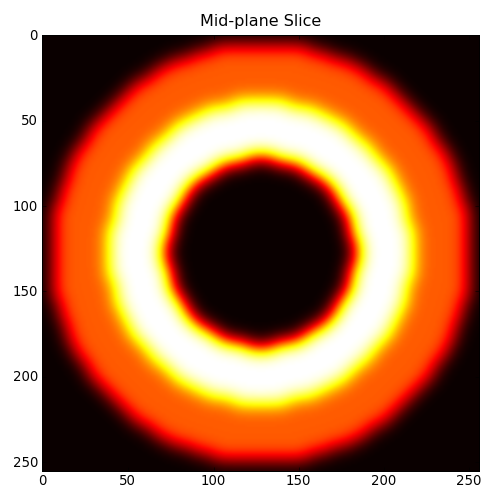## Three Spheres

• Regular Grid in .raw/.nrrd format. Spheres.zip
• Datatype = Float
• Dimensions = 256 256 256
• File Size = 50 MB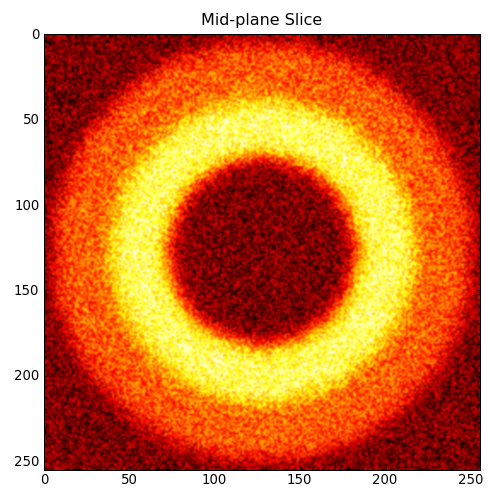## Three Spheres with Gaussian Noise

• Regular Grid in .raw/.nrrd format. Noisy_spheres.zip
• Datatype = Float
• Dimensions = 256 256 256
• File Size = 58 MB

## Standard Datasets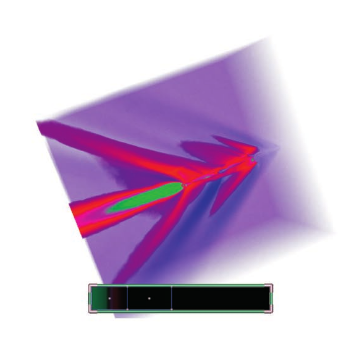## Fighter

• Unstructured Tet-Mesh in .off format. Fighter.off
• Datatype = Float
• Num Vertex = 256,614
• Num Tets = 1,403,504
• File Size = 48 MB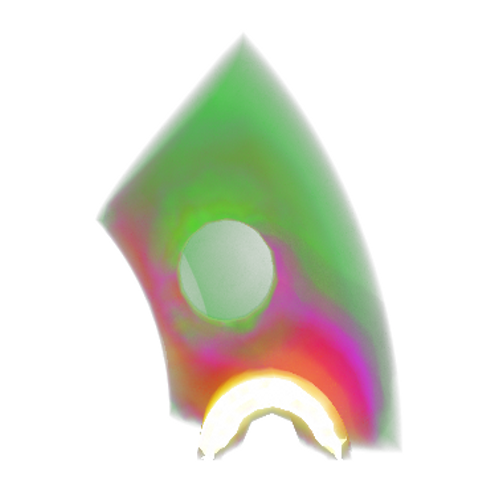## SPX

• Unstructured Tet-Mesh in .off format. Spx.off
• Datatype = Float
• Num Vertex = 20,108
• Num Tets = 12,936
• File Size = 993 KB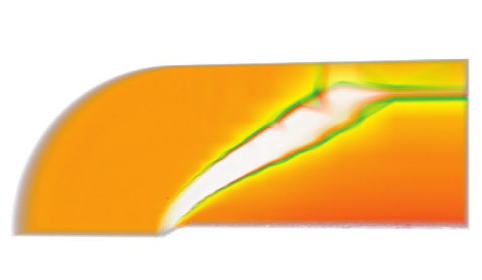## Blunt Fin

• Unstructured Tet-Mesh in .off format. Blunt.off
• Datatype = Float
• Num Vertex = 40,960
• Num Tets = 187,395
• File Size = 5.8 MB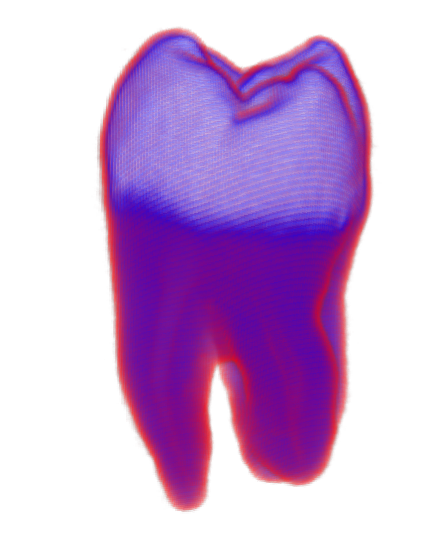## CT-Tooth

• Regular Grid in .raw/.nrrd format. Tooth.zip
• Datatype = Float
• Dimensions = 103, 94, 161
• File Size = 2.0 MB

## Collaborator-generated Data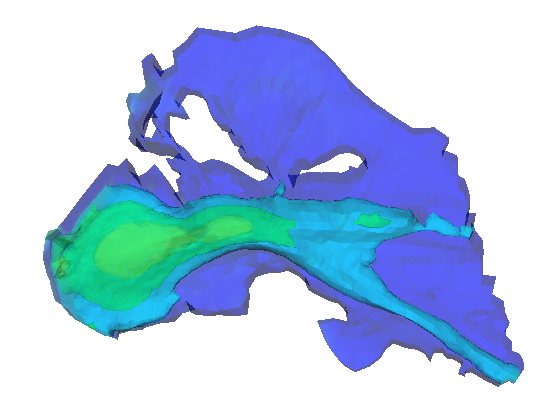## Columbia River Simulation: Thanks to Antonio Baptista (STCCMOP)

• Unstructured Prism Mesh converted to .vtk format. Inlet.vtk
• Num Vertex = 33,410
• Num Prisms = 57,825
• File Size = 4.4 MB
• Scalar Fields
• salt - salinity field
• vpos - vertical position
• h - bathymetry
• saltflux - salinity flux
• Vector Fields
• uv - horizontal current velocities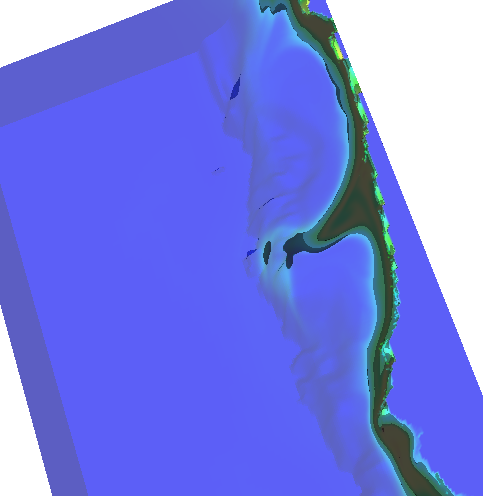## Coastal Ocean Simulation: Thanks to Yvette Spitz (STCCMOP)

• Curvilinear Grid converted to .vtk format. Coastal.vtk
• Dimensions = 384, 128, 44
• File Size = 25 MB
• Scalar Fields
• phytoplankton - Phytoplankton Concentration
• zooplankton - Zooplankton Concentration
• NO3 - Nitrate Level
• temperature - Temperature (Degrees Celsius)
• Vector Fields
• velocity - 3D Water Velocity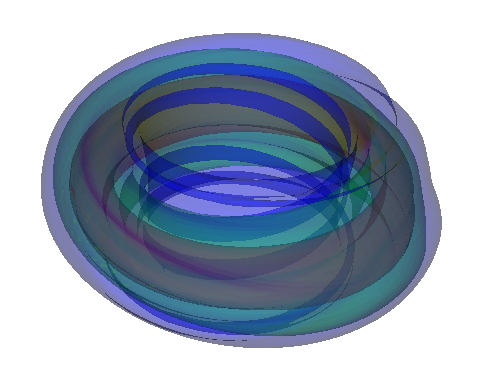## Tokamak Fusion: Thanks to Allen Sanderson (SDM)

• Unstructured Hex Mesh converted to .vtk format. Tokamak.vtk
• Num Vertex = 622,261
• Num Hexes = 600,000
• File Size = 59 MB
• Scalar Field
• scalars - Temperature (Degrees Kelvin)
• Vector Field
• vectors - Magnetic Field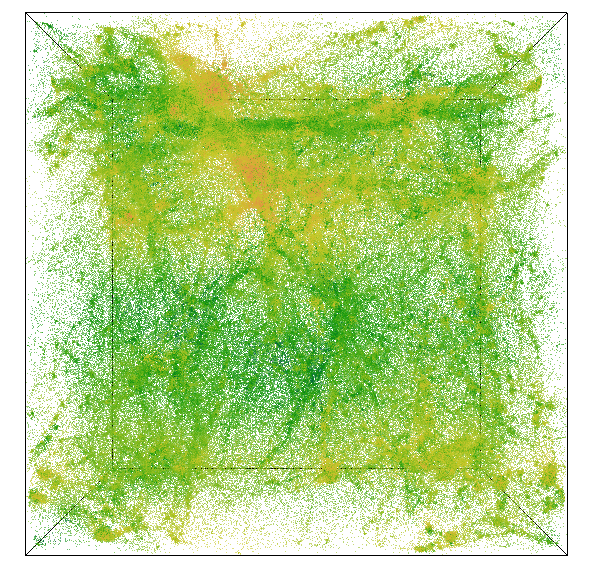## Cosmology Simulation: Thanks to Katrin Heitmann (LANL)

• Point cloud converted to .vtk format. Cosmology.vtk
• Num Points = 1,048,576
• File Size = 45 MB
• Scalar Field
• mass - Mass (Solar Masses)
• tag - Id
• Vector Field
• velocity - 3D velocity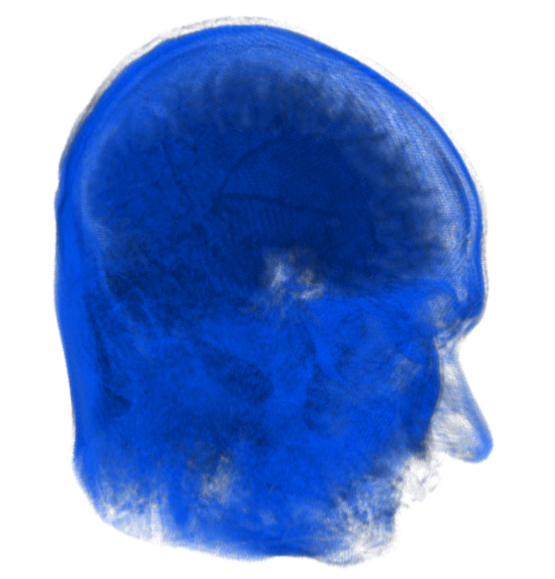## Magnetic Resonance Image: Thanks to John Schreiner

• Regular Grid converted to .vtk format. Mri.vtk
• Dimensions = 256, 256, 208
• Datatype = Unsigned Short
• File Size = 27 MB